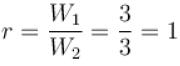Courses

# Statistical Physics NAT Level – 2

## 10 Questions MCQ Test Topic wise Tests for IIT JAM Physics | Statistical Physics NAT Level – 2

Description
This mock test of Statistical Physics NAT Level – 2 for IIT JAM helps you for every IIT JAM entrance exam. This contains 10 Multiple Choice Questions for IIT JAM Statistical Physics NAT Level – 2 (mcq) to study with solutions a complete question bank. The solved questions answers in this Statistical Physics NAT Level – 2 quiz give you a good mix of easy questions and tough questions. IIT JAM students definitely take this Statistical Physics NAT Level – 2 exercise for a better result in the exam. You can find other Statistical Physics NAT Level – 2 extra questions, long questions & short questions for IIT JAM on EduRev as well by searching above.
*Answer can only contain numeric values
QUESTION: 1

### The pressure for a non-interacting Fermi gas with internal energy U at temperature T is given as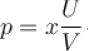. Find value of x?

Solution:

The mean pressure of the perfect Fermi gas can be written as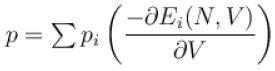The average energy of the system is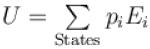For an ideal fermion is a cubical box, the energy of single particle states are given by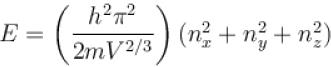If there is no interaction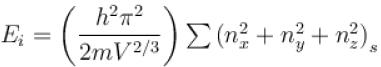Ei = energy of ith microstate of the system.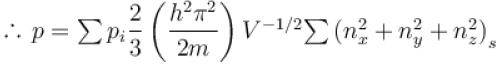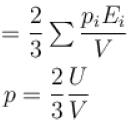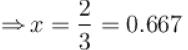*Answer can only contain numeric values
QUESTION: 2

### Boltzmann distribution of particles between different states under equilibrium at temperature T is given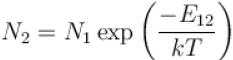Vibrational states have equal spacing hv as a first approximation. For a particular case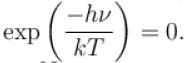Out of N molecules, a number N0 is in the ground state. Then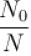is nearest to?

Solution: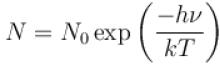= N0 (1 + 0.4 + 0.42 + ...)
N = N0 (1.68) nearly
⇒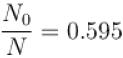*Answer can only contain numeric values
QUESTION: 3

### According to Fermi-Dirac statistic the number of particles in a phase cell is.

Solution:

Systems following Fermi-Dirac statistics obey Pauli’s exclusion principal and each phase cell corresponding to one energy level which can be occupied by only 1 particle.

*Answer can only contain numeric values
QUESTION: 4

Six distinguishable particles are distributed over three non-degenerate levels of energies 0, E and 2E. The total energy of the distribution for which the probability is a maximum is (in terms of E)?

Solution:

Since, the levels are non-degenerate there is only one state associated with each energy.
Let the number of particles is 3 energy state be N1 , N2 and N3 respectively.
where N1 + N2 + N3 = 6
As the particles are distinguishable, the number of microstates i.e. the number of ways of choosing N1 , N2 and N3 particles from 6 particles is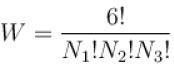W →  thermodynamic probability. It is maximum when N1 !N2 !N3 ! is a minimum
where N1 = N2 = N3 = 2
The corresponding total energy of distribution is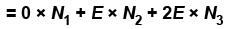= 6E

*Answer can only contain numeric values
QUESTION: 5

Consider a radiation cavity of volume V at temperature T. The average number of photons in equilibrium is proportional to Tα , Find α?

Solution:

For photons, B-E statistics is applicable
For photons,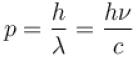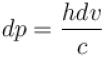the density of states for photons between p and p + dp is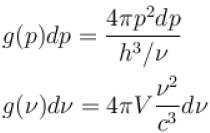Since two independent direction of polarization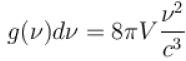hv = dE
⇒ hdv = dE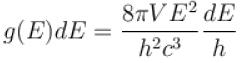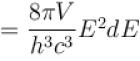Average number of photons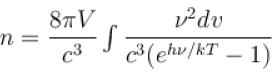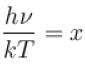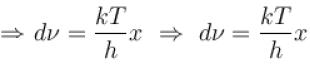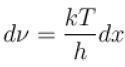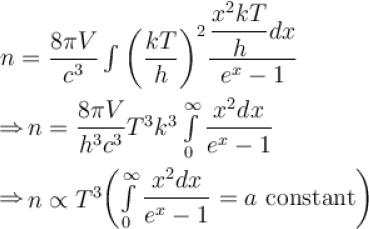*Answer can only contain numeric values
QUESTION: 6

Ratio is average energy of an electron in a metal at T = 0 to the Fermi energy at T = 0 is.

Solution:

The total number of cells upto energy level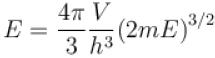Thus, the number of cells corresponding to energy interval E and E + dE is given by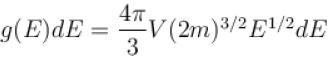The total number of cells between energy level 0 and EF at T = 0, are equal to the total number of particles i.e.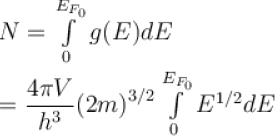Thus,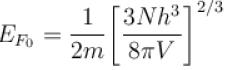Efo is value of EF at T = 0.
The average kinetic energy of the particle at T = 0 is given by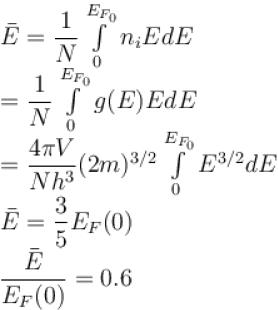*Answer can only contain numeric values
QUESTION: 7

Consider a system of 2 identical particles each of which can be in any one of the 3 single particles states. The number states of the systems are possible in B-E statistics?

Solution:

The total number of way W, of distributing 2 identical particles in the given system is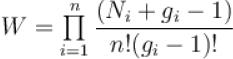ni = 2, gi = 2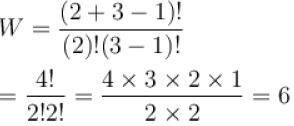*Answer can only contain numeric values
QUESTION: 8

The probability that a state which is 0.2eV above the Fermi energy in metal atom at 700K is.

Solution:

Probability of occupancy of a given state with energy E is given by the FermiDirac distribution formula which is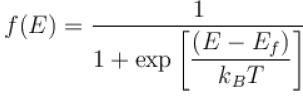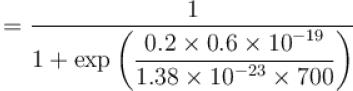f(E) = 0.035

*Answer can only contain numeric values
QUESTION: 9

The Fermi energy of a free electron gas depends on the electron density ρ as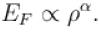Find the value of α?

Solution:

The Fermi energy of the electron gas is given by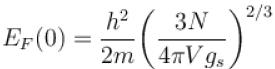with gs = 2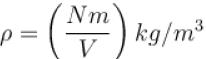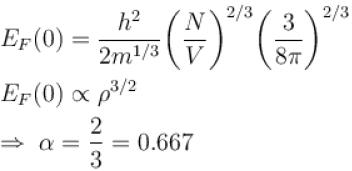*Answer can only contain numeric values
QUESTION: 10

In a system of particles, each particles can be in any one of three possible quantum states. The ratio of the probability that the two particles occupy the same state to the probability that the two particle occupy different state for B-E statistics is.

Solution:

For B-E statististic, the particles are indistinguishable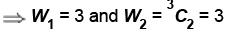So,